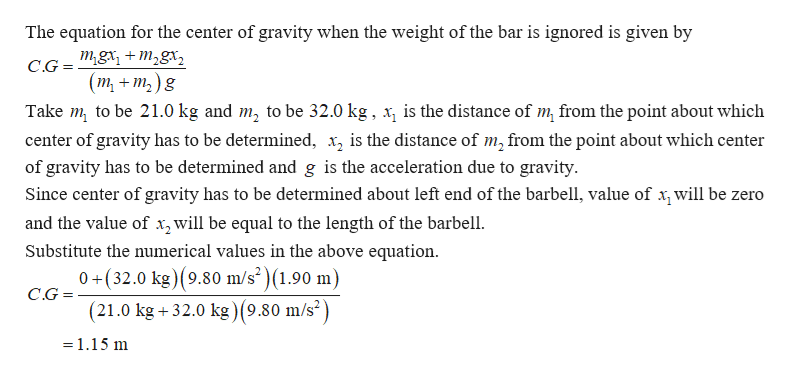# A 1.90-m-long barbell has a 21.0 kg weight on its left end and a 32.0 kg weight on its right end.If you ignore the weight of the bar itself, how far from the left end of the barbell is the center of gravity?Express your answer to three significant figures and include the appropriate units. Where is the center of gravity if the 9.00 kg mass of the barbell itself is taken into account?Express your answer to three significant figures and include the appropriate units.

Question
48 views

A 1.90-m-long barbell has a 21.0 kg weight on its left end and a 32.0 kg weight on its right end.

If you ignore the weight of the bar itself, how far from the left end of the barbell is the center of gravity?
Express your answer to three significant figures and include the appropriate units.

Where is the center of gravity if the 9.00 kg mass of the barbell itself is taken into account?
Express your answer to three significant figures and include the appropriate units.
check_circle

Step 1help_outlineImage TranscriptioncloseThe equation for the center of gravity when the weight of the bar is ignored is given by mgrm2gx + C.G = (т, + т,)g Take m to be 21.0 kg and m2 to be 32.0 kg, is the distance of m from the point about which center of gravity has to be determined, x2 is the distance of m2 from the point about which center of gravity has to be determined and g is the acceleration due to gravity Since center of gravity has to be determined about left end of the barbell, value of x,will be zero and the value of x^will be equal to the length of the barbell Substitute the numerical values in the above equation 0+(32.0 kg) (9.80 m/s)(1.90 m) C.G= (21.0 kg +32.0 kg) (9.80 m/s2) =1.15 m fullscreen

### Want to see the full answer?

See Solution

#### Want to see this answer and more?

Solutions are written by subject experts who are available 24/7. Questions are typically answered within 1 hour.*

See Solution
*Response times may vary by subject and question.
Tagged in

### Physics